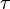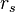# Schwarschild metric

In Einstein’s theory of general relativity, the Schwarzschild metric (also known as the Schwarzschild vacuum or Schwarzschild solution) is a solution to the Einstein field equations which describes thegravitational field outside a spherical mass, on the assumption that the electric charge of the mass, angular momentum of the mass, and universal cosmological constant are all zero. The solution is a useful approximation for describing slowly rotating astronomical objects such as many stars and planets, including Earth and the Sun. The solution is named after Karl Schwarzschild, who first published the solution in 1916.

According to Birkhoff’s theorem, the Schwarzschild metric is the most general spherically symmetric, vacuum solution of the Einstein field equations. A Schwarzschild black hole or static black hole is a black hole that has no charge or angular momentum. A Schwarzschild black hole is described by the Schwarzschild metric, and cannot be distinguished from any other Schwarzschild black hole except by its mass.

The Schwarzschild black hole is characterized by a surrounding spherical surface, called the event horizon, which is situated at the Schwarzschild radius, often called the radius of a black hole. Any non-rotating and non-charged mass that is smaller than its Schwarzschild radius forms a black hole. The solution of the Einstein field equations is valid for any mass M, so in principle (according to general relativity theory) a Schwarzschild black hole of any mass could exist if conditions became sufficiently favorable to allow for its formation.

## The Schwarzschild metric

In Schwarzschild coordinates, the line element for the Schwarzschild metric has the form$c^2 {d tau}^{2} = left(1 - frac{r_s}{r} right) c^2 dt^2 - left(1-frac{r_s}{r}right)^{-1} dr^2 - r^2 left(dtheta^2 + sin^2theta , dvarphi^2right),$

where

•$tau$ is the proper time (time measured by a clock moving along the same world line with the test particle),
• c is the speed of light,
• t is the time coordinate (measured by a stationary clock located infinitely far from the massive body),
•$r$ is the radial coordinate (measured as the circumference, divided by 2π, of a sphere centered around the massive body),
• θ is the colatitude (angle from North, in units of radians),
• φ is the longitude (also in radians), and
•$r_s$ is the Schwarzschild radius of the massive body, a scale factor which is related to its mass M by rs = 2GM/c2, where G is the gravitational constant.

The analogue of this solution in classical Newtonian theory of gravity corresponds to the gravitational field around a point particle.

In practice, the ratio rs/r is almost always extremely small. For example, the Schwarzschild radius rs of the Earth is roughly 8.9 millimeters (0.35 in), while the sun, which is 3.3×105 times as massive has a Schwarzschild radius of approximately 3.0 km (1.9 mi). Even at the surface of the Earth, the corrections to Newtonian gravity are only one part in a billion. The ratio only becomes large close to black holes and other ultra-dense objects such asneutron stars.

The Schwarzschild metric is a solution of Einstein’s field equations in empty space, meaning that it is valid only outside the gravitating body. That is, for a spherical body of radius R the solution is valid for r > R. To describe the gravitational field both inside and outside the gravitating body the Schwarzschild solution must be matched with some suitable interior solution at r = R.

Source: Wikipedia